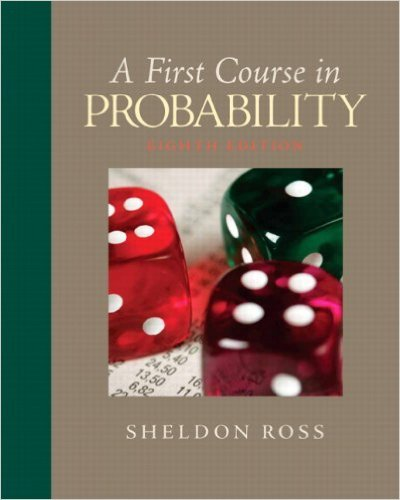×
×

# Solutions for Chapter 9: First Course in Probability 8th Edition## Full solutions for First Course in Probability | 8th Edition

ISBN: 9780136033134Solutions for Chapter 9

Solutions for Chapter 9
4 5 0 285 Reviews
29
2
##### ISBN: 9780136033134

Since 11 problems in chapter 9 have been answered, more than 5879 students have viewed full step-by-step solutions from this chapter. First Course in Probability was written by and is associated to the ISBN: 9780136033134. Chapter 9 includes 11 full step-by-step solutions. This expansive textbook survival guide covers the following chapters and their solutions. This textbook survival guide was created for the textbook: First Course in Probability, edition: 8.

Key Statistics Terms and definitions covered in this textbook
• Acceptance region

In hypothesis testing, a region in the sample space of the test statistic such that if the test statistic falls within it, the null hypothesis cannot be rejected. This terminology is used because rejection of H0 is always a strong conclusion and acceptance of H0 is generally a weak conclusion

A variation of the R 2 statistic that compensates for the number of parameters in a regression model. Essentially, the adjustment is a penalty for increasing the number of parameters in the model. Alias. In a fractional factorial experiment when certain factor effects cannot be estimated uniquely, they are said to be aliased.

• All possible (subsets) regressions

A method of variable selection in regression that examines all possible subsets of the candidate regressor variables. Eficient computer algorithms have been developed for implementing all possible regressions

• Arithmetic mean

The arithmetic mean of a set of numbers x1 , x2 ,…, xn is their sum divided by the number of observations, or ( / )1 1 n xi t n ? = . The arithmetic mean is usually denoted by x , and is often called the average

• Assignable cause

The portion of the variability in a set of observations that can be traced to speciic causes, such as operators, materials, or equipment. Also called a special cause.

• Asymptotic relative eficiency (ARE)

Used to compare hypothesis tests. The ARE of one test relative to another is the limiting ratio of the sample sizes necessary to obtain identical error probabilities for the two procedures.

• Average run length, or ARL

The average number of samples taken in a process monitoring or inspection scheme until the scheme signals that the process is operating at a level different from the level in which it began.

• Bivariate normal distribution

The joint distribution of two normal random variables

• Continuous distribution

A probability distribution for a continuous random variable.

• Correlation matrix

A square matrix that contains the correlations among a set of random variables, say, XX X 1 2 k , ,…, . The main diagonal elements of the matrix are unity and the off-diagonal elements rij are the correlations between Xi and Xj .

• Counting techniques

Formulas used to determine the number of elements in sample spaces and events.

• Crossed factors

Another name for factors that are arranged in a factorial experiment.

• Defect concentration diagram

A quality tool that graphically shows the location of defects on a part or in a process.

• Error sum of squares

In analysis of variance, this is the portion of total variability that is due to the random component in the data. It is usually based on replication of observations at certain treatment combinations in the experiment. It is sometimes called the residual sum of squares, although this is really a better term to use only when the sum of squares is based on the remnants of a model-itting process and not on replication.

• False alarm

A signal from a control chart when no assignable causes are present

• Finite population correction factor

A term in the formula for the variance of a hypergeometric random variable.

• First-order model

A model that contains only irstorder terms. For example, the irst-order response surface model in two variables is y xx = + ?? ? ? 0 11 2 2 + + . A irst-order model is also called a main effects model

• Fraction defective

In statistical quality control, that portion of a number of units or the output of a process that is defective.

• Fractional factorial experiment

A type of factorial experiment in which not all possible treatment combinations are run. This is usually done to reduce the size of an experiment with several factors.

• Generator

Effects in a fractional factorial experiment that are used to construct the experimental tests used in the experiment. The generators also deine the aliases.

×